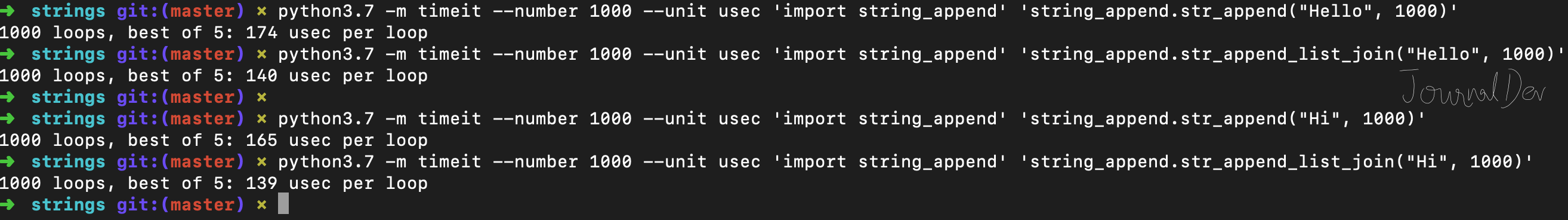# Python String Append

Published on August 3, 2022By Pankaj
Developer and author at DigitalOcean.While we believe that this content benefits our community, we have not yet thoroughly reviewed it. If you have any suggestions for improvements, please let us know by clicking the “report an issue“ button at the bottom of the tutorial.

Python string object is immutable. So every time we use + operator to concatenate two strings, a new string is created. If we have to append many strings, using + operator will unnecessarily create many temporary strings before we have the final result.

## Python String Append

Let’s look at a function to concatenate a string ‘n’ times.

``````def str_append(s, n):
output = ''
i = 0
while i < n:
output += s
i = i + 1
return output
``````

Note that I am defining this function to showcase the usage of + operator. I will later use timeit module to test the performance. If you simply want to concatenate a string ‘n’ times, you can do it easily using `s = 'Hi' * 10`.

Another way to perform string append operation is by creating a list and appending strings to the list. Then use string join() function to merge them together to get the result string.

``````def str_append_list_join(s, n):
l1 = []
i = 0
while i < n:
l1.append(s)
i += 1
return ''.join(l1)
``````

Let’s test these methods to make sure they are working as expected.

``````if __name__ == "__main__":
print('Append using + operator:', str_append('Hi', 10))
print('Append using list and join():', str_append_list_join('Hi', 10))
# use below for this case, above methods are created so that we can
# check performance using timeit module
print('Append using * operator:', 'Hi' * 10)
``````

Output:

``````Append using + operator: HiHiHiHiHiHiHiHiHiHi
Append using list and join(): HiHiHiHiHiHiHiHiHiHi
Append using * operator: HiHiHiHiHiHiHiHiHiHi
``````

## Best way to append strings in Python

I have both the methods defined in `string_append.py` file. Let’s use timeit module to check their performance.

``````\$ python3.7 -m timeit --number 1000 --unit usec 'import string_append' 'string_append.str_append("Hello", 1000)'
1000 loops, best of 5: 174 usec per loop
\$ python3.7 -m timeit --number 1000 --unit usec 'import string_append' 'string_append.str_append_list_join("Hello", 1000)'
1000 loops, best of 5: 140 usec per loop

\$ python3.7 -m timeit --number 1000 --unit usec 'import string_append' 'string_append.str_append("Hi", 1000)'
1000 loops, best of 5: 165 usec per loop
\$ python3.7 -m timeit --number 1000 --unit usec 'import string_append' 'string_append.str_append_list_join("Hi", 1000)'
1000 loops, best of 5: 139 usec per loop
``````## Summary

If there are few strings, then you can use any method to append them. From a readability perspective, using + operator seems better for few strings. However, if you have to append a lot of string, then you should use the list and join() function.

You can checkout complete python script and more Python examples from our GitHub Repository.Pankaj

author

Developer and author at DigitalOcean.

#### Still looking for an answer?

from datetime import datetime start_time=datetime.now().strftime(“%H:%M”) flight_time=13.0987minutes k=start_time+flight time I need to add the current time and flight time could you please help me withs this?

- swathi meka